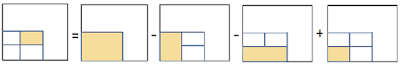## Wednesday, 6 November 2013

### Summed Area Tables (Integral Images), Explanation and Implementation in C++

In 1984, Crow proposed an image representation where each pixel value is replaced by the sum of all the pixels that belong to the rectangle defined by the lower left corner of the image and the pixel of our interest .
Even though more storage space may be required to save the image, the sum of every rectangle in the image can be calculated in constant time once the table is constructed. The summed area table can be constructed in linear time O(n), where n is the number of pixels in the image, since one iteration through the entire image is enough to replace the pixel values inside the area table.Figure 1. This figure depicts the parameters of the following equation .

So, let’s assume the we have the above image and we would like to find the sum of the blue area defined by the pixels (x,y) and (x+lenX, y+lenY) included. Then the sum is given by:
sum =  T(x+lenX, y+lenY) - T(x+lenX, y-1) - T(x-1,y+lenY) +  T(x-1, y-1)Figure 2. Once the Integral Image is constructed, the sum of any rectangular area is calculated in constant time .

Where T(x,y) is the value in the table with coordinates (x,y).

Code Available here:
https://www.dropbox.com/s/83oynkf11rrjd21/SumTables.zip

The images are taken from the following article, which explains how Sum Area Tables, in other words Integral Images, are used in 3D (named Integral Volumes) to optimise reconstruction of polygon representations from voxelised data: https://www.mdpi.com/2072-4292/13/4/559

Work Cited

 Crow, F.C. (1984, July). Summed-Area Tables for Texture Mapping. ACM, Computer Graphics, Volume 18, Number 3

 Miltiadou M, Campbell NDF, Cosker D, Grant MG. A Comparative Study about Data Structures Used for Efficient Management of Voxelised Full-Waveform Airborne LiDAR Data during 3D Polygonal Model Creation. Remote Sensing. 2021; 13(4):559. https://doi.org/10.3390/rs13040559

1.if(i_posX==0)
{
return m_values[getIndex(i_lenX,i_posY+i_lenY)]
-m_values[getIndex(i_posX,i_posY-1)];
}

Must be:

if(i_posX==0)
{
return m_values[getIndex(i_lenX,i_posY+i_lenY)]
-m_values[getIndex(i_lenX,i_posY-1)];
}

1.Thanks for the corrections and sorry about the late reply. You are right. =)

2.This comment has been removed by the author.